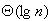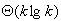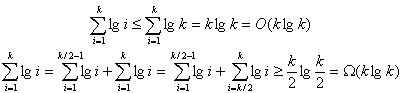# Implementation of an Efficient Priority Queue for a UNIX Scheduler

## CS 161Programming Project

### Richard Bragg

The Data Structure

After some careful analysis of which data structure to use for this project, I chose to implement my scheduler using a binary heap stored as an array [CLRS Chapter 6].  While the actual data structure itself is identical to the binary heap data structure discussed in CLRS, I did change the operations slightly to increase the overall efficiency of the heap to be used as a real priority queue.

The first change is in the Extract-Max function - rather than extracting the maximum node from the heap, then fixing the remaining nodes to maintain the heap property, I extract the maximum node and simply mark the heap as invalid.  Any heap operations that are invoked on a "valid" heap proceed as they would normally.  However, if the Insert operation is invoked on an "invalid" heap, then the new node is inserted at the newly vacated top position on the heap and moved down the heap to a legal position using a call to Heapify.  For all commands besides Insert called on an "invalid" heap, the heap must first be "fixed" using the same procedure that Extract-Max would typically use, then the algorithms proceed as usual.  The large gain here is obviously found only when an Insert call follows an Extract-Max call.  Under the normal implementation, a call to Extract-Max would return the top element in the heap, swap the bottom element in the heap to the top position, and then call Heapify from the top position - an O(lg n) operation.  If a call to Insert came next, the element would be added at the bottom of the heap and moved up to a legal position using Increase-Key - another O(lg n) operation.  Clearly, in my implementation, we eliminate the need for one of these two O(lg n) operations in the situation in which Insert follows Extract-Max.  This algorithmic improvement is key in the UNIX scheduler, since quite often a node is extracted and then re-inserted.

The second change is also in the Extract-Max function, and is really a byproduct of the first change.  Rather than dynamically allocating memory and making a copy of the node during Extract-Max, I can simply pass a pointer to the first node in the "invalid" heap with the stipulation that if the client wants to keep the node, it must make its own copy before calling any other function on the priority queue.  Since the scheduler only needs to look at the values in the maximum node, it is never necessary to make this copy, and we gain the time that would have been required for allocating the memory, copying the node, and freeing the memory using this method.

The Operations

Interface Methods

• Insert(K, I) - If the heap is "valid", add the new node at the last position in the heap and move it up to a legal position using Increase-Key [CLRS].  If the heap is "invalid", add the new node at the first position in the heap and move it down to a legal position using Heapify [CLRS].  The result of this operation is a "valid" heap.
• Delete(E) - If the heap is "invalid", first fix it using Fix_Heap.   Then swap the last node of the array into the position of the deleted node and move it down to a legal position using Heapify [CLRS].  The result of this operation is a "valid" heap.
• Change-Priority(E,K) - If the heap is "invalid", first fix it using Fix-Heap.  Then compare the specified node with its parent:  if the parent is greater, move the node down to a legal position using Heapify [CLRS].  If the parent is less, move the node up to a legal position using Increase-Key [CLRS].  The result of this operation is a "valid" heap. (NOTE: I include this rather than Increase-Key, as specified in the handout, since this is actually the function that is called by the scheduler, and Increase-Key is implemented only as a helper function.  The function I describe here is actually more general than Increase-Key.)
• Maximum() - If the heap is "invalid", first fix it using Fix-Heap.  Then simply return a pointer to the first node in the heap.  The result of this operation is a "valid" heap.
• Extract-Max() - If the heap is "invalid", first fix it using Fix-Heap.  Then simply return a pointer to the first node in the heap, but mark the heap as "invalid".  Since the node is not copied and will only exist until Fix-Heap is called, the client must make its own copy of the node for it to be valid once another operation is invoked.  The result of this operation is an "invalid" heap, in which there are (heapsize + 1) elements in the heap and the first node in the heap is technically no longer a member of the priority queue and will be deleted on the next call to any of the operations.
Helper Methods
• Fix-Heap() - Simply move the last node of the (heapsize + 1) "invalid" heap resulting from Extract-Max to the first position in the array and move it down to a legal position using Heapify [CLRS].  This operation always results in a "valid" heap.
• Increase-Key(E) - Implemented exactly as described in CLRS.
• Heapify(E) - Implemented exactly as described in CLRS.

Running Time

Per-Operation Basis
Since any of the operations could be called on an "invalid" heap, a call to either Heapify or Increase-Key could be required for any of the 5 basic operations - either directly in the case of Insert, or indirectly via a call to Fix-Heap in the other 4 operations.  Since the asymptotic running times for both Heapify and Increase-Key are bounded from above by O(lg n) where n is the number of nodes in the queue [CLRS], all operations must have per-operation running times of at least O(lg n).  It makes more sense then, to use an amortized analysis here, since the Fix-Heap operation is only necessary immediately after a call to Extract-Max.

Amortized Analysis
The important insight to make here is that when Extract-Max is called, it must pay an extra O(lg n) to account for the fact that it leaves the heap in an "invalid" state, and it will need that O(lg n) to return the heap to a "valid" state.  Thus whenever any of the other operations are called, we can ignore the cost of fixing the heap since it was paid in advance by Extract-Max.

• Insert(K, I) - The running time of this one depends on whether or not the heap is "invalid".  If the heap is "valid", it will cost O(lg n) to add the new node and move it the maximum of O(lg n) levels up the heap.  If the heap is "invalid", it can use the O(lg n) paid in advance by Extract-Max to pay for the O(lg n) needed to add the new node and move it the maximum of O(lg n) positions down the heap - thus in this case it is O(1). Result: O(lg n) amortized cost on a valid heap, O(1) amortized cost on an invalid heap
• Delete(E) - If the heap is "invalid", it can use the O(lg n) paid in advance by Extract-Max to call Fix_Heap.   The resulting operation costs an additional O(lg n), since the node that is swapped into the position of the deleted node can move up to the maximum of O(lg n) positions down the heap. Result: O(lg n) amortized cost
• Change-Priority(E,K) - If the heap is "invalid", it can use the O(lg n) paid in advance by Extract-Max to call Fix_Heap.   The resulting operation costs an additional O(lg n), since the node whose priority is changed can move up to the maximum of O(lg n) positions up or down the heap based on its new priority. Result: O(lg n) amortized cost
• Maximum() - If the heap is "invalid", it can use the O(lg n) paid in advance by Extract-Max to call Fix_Heap.   The resulting operation is only an additional O(1), since it simply returns a pointer. Result: O(1) amortized cost
• Extract-Max() - If the heap is "invalid", it can use the O(lg n) paid in advance by the previous call to Extract-Max to call Fix_Heap.   But then once again, this must pay the O(lg n) in advance for the heap to be fixed by the next call. Result: O(lg n) amortized cost
Since the Insert operation is now only O(1) in some cases, clearly this is more efficient over a sequence of operations than the standard implementation of binary heaps presented in CLRS.

Best Case Input

The best case input would be any sequence of operations in which each of the operations were only called under the following conditions:

• Insert(K, I) - This could be called either immediately following a call to Extract-Max with the inserted node greater than all of the nodes already in the heap, or it could on a "valid" heap with the inserted node less than all of the nodes already in the heap, so that in either case the inserted node would not have to move from the position in which it was inserted.  This best case running time would be O(1) actual cost.
• Delete(E) - This could not follow Extract-Max, and could only be called on the last node in the heap at that time, so that we could delete the node simply by decreasing the heapsize by 1. This best case running time would be O(1) actual cost.
• Change-Priority(E,K) - This could not follow Extract-Max, and could only be called in such a way that the new priority of the changed node would not have to change its position in the heap - in other words its new priority must still be less than its parent and greater than its children. This best case running time would be O(1) actual cost.
• Maximum() - This can be called at any time, except after Extract-Max. This best case running time would be O(1) actual cost.
• Extract-Max() - This can be called at any time, provided that the next operation called is an Insert operation, subject to the constraints listed for that operation. This best case running time would be O(1) actual cost.
Since each of the 5 operations can be run in O(1) actual time in the best case, a sequence of any k operations subject to these constraints could be run inactual time in the best case.

Worst Case Input

The worst case input for each operation would involve the operations being called under the following conditions:

• Insert(K, I) - This could never be called following Extract-Max (thus avoiding the associated gain with that combination), and the inserted node must always be greater than every node already in the heap, causing the node to travel from position at the bottom of the heap all the way to the top position in the heap.  This worst case running time would beamortized cost.
• Delete(E) - This must always follows Extract-Max, and must always be called on the first node in the heap at that time, so that the node that replaces it from the bottom of the heap must travel the maximum distance back down the heap.  This worst case running time would beamortized cost.
• Change-Priority(E,K) - This must always follows Extract-Max, and must always be called on the first node in the heap at that time with a priority that is less than all the nodes in the heap, OR on the last node in the heap at that time with a priority that is greater than all the nodes in the heap, so that in either case the node must travel the maximum distance up or down the heap to find a legal position.  This worst case running time would beamortized cost.
• Maximum() - This has a worst case amortized cost of O(1), so there is no difference between its best and worst case.
• Extract-Max() - This can be called at any time, provided that the next operation called is not an Insert operation, so that the full effect of theamortized cost is felt.
The worst case running time of a sequence of k operations would be a sequence of k calls to Insert where each consecutive node inserted was larger than the previous one.  Only calls to Insert would be included in this worst case, since that is the only operation that increases the size of the priority cue, and thus is the only way to make a single operation take more time than the call before it.  This worst case running time on k operations would take, with the derivation as follows:Comparison to Other Data Structures

In choosing the best data structure to use from the list we were given, I made the following table to aid in my decision, where I include my solution for comparison.

Comparison of Candidate Data Structures

 Insert(K,I) Delete(E) Increase-Key(E,K) Maximum() Extract-Max() Other considerations Doubly Linked Lists O(n) average/worst case O(1) O(n) average/worst case O(1) O(1) data structure to beat for this assignment Binary Heaps O(lg n) average/worst case O(lg n) average/worst case O(lg n) average/worst case O(1) O(lg n) average/worst case efficient, simple to implement and debug Binomial Queues O(lg n) worst case O(lg n) average/worst case O(lg n) average/worst case O(1) O(lg n) average/worst case difficult to implement, main advantage  is the Union operation [CLRS] Leftist Trees O(lg n) O(1) to mark the node only, deleted later O(lg n) O(1) O(lg n) 30% slower than standard heaps [Jones86] Pagodas O(n) worst case for some operations, complicated implementation [Mhatre01] Fibonacci Heaps O(1) amortized O(lg n) amortized O(1) amortized O(1) amortized O(lg n) amortized best when Extract-Max and Delete are called infrequently [CLRS], better  for large queue sizes Modified Binary Heaps (My solution) O(1) on invalid heap, O(lg n) on valid heap (amortized) O(lg n) amortized O(1) amortized O(1) amortized O(lg n) amortized simple but efficient, especially when Extract-Max is followed by Insert

The textbook [CLRS] and [Mhatre01] were of tremendous help in filling in this table.  While searching for algorithms, I was looking for a data structure that was relatively straightforward to implement and debug (since I was working by myself), but also performed well compared to the other alternatives.  After reviewing all the other data structures, I determined that the simple binary heaps represented the ideal tradeoff between performance and ease of implementation.  It also provided the benefit of only requiring the memory to be allocated once (provided we know the maximum heap size), and once it was created all of the operations could be performed in place.  Once I noticed I could even improve the performance of binary heaps for the purpose of the scheduler using the modifications I have presented here, I felt I had been able to create a solution that was on par with the best from the list above!

Real-Time Analysis

The development of the algorithm presented here was not developed all at once.  It took a series of improvements.  First I implemented a binary heap exactly as it appeared in CLRS (Rev. 1).  Then I noticed that I could modify the algorithm to defer fixing the heap until the call following Extract-Max, since so often it was following by an Insert (Rev. 2).  Finally, I noticed that it was unnecessary to copy the node for the client in Extract-Max, since it was only needed temporarily by the scheduler (Rev. 3).  In Rev. 3, I also optimized the code itself as much as possible, using pointer arithmetic instead of array accesses and other such speed-ups I have learned over the years.  In the end, I was able to develop an implementation that equaled or outperformed the supplied doubly linked list in every case, including the test file "test.10000.a", which was supposedly one of the best case performances of that implementation.  While my implementation required slightly more comparisons for that input file, the fact that no memory needed to be allocated in my implementation probably made up the difference.  The following table shows the evolution of my project (all tests were performed on elaine27 and averaged over 5 trials).  Rev. 3 is what I have submitted with this report, and clearly shows a significant improvement from both the doubly linked list, and just the basic binary heap as defined in CLRS.

Real-Time Performance Improvements of the Binary Heap Implementation
(Total Time (seconds) / Client Time (seconds) / Comparisons)

 Test File Doubly Linked List  (Sample Implemenation) Binary Heap (Rev. 1) Binary Heap (Rev. 2) Binary Heap (Rev. 3) test.100.a 1.128 / 0.102 / 967,642 0.427 / 0.107 / 234,228 0.398 / 0.095 / 211,467 0.358 / 0.069 / 211,467 test.100.b 1.139 / 0.101 / 968,434 0.427 / 0.111 / 234,388 0.396 / 0.099 / 211,308 0.359 / 0.084 / 211,308 test.500.a 31.17 / 2.61 / 27,787,093 3.426 / 0.790 / 1,957,773 3.254 / 0.818 / 1,851,882 3.08 / 0.666 / 1,851,882 test.500.a.rev 31.01 / 2.64 / 27,781,669 3.428 / 0.804 / 1,955,706 3.304 / 0.782 / 1,850,521 3.088 / 0.610 / 1,850,521 test.1000.a 168.47 / 12.93 / 151,071,987 10.632 / 2.620 / 6,097,461 10.406 / 2.550 / 5,855,713 9.776 / 1.916 / 5,855,713 test.10000.a 3.068 / 0.634 / 1,206,286 5.332 / 1.456 / 2,228,595 3.819 / 1.01 / 1,460,753 3.064 / 0.632 / 1,460,753

References

[CLRS] Cormen, T.H, Leiserson, C.E., Rivest, R.L., and Stein, C.  Introduction to Algorithms, Second Edition.  McGraw Hill, 2001.
[Jones86] Jones, D.W., An Empirical Comparison of Priority-Queue and Event-Set-Implementations. Communications of the ACM, 1986. 29(4): p. 300-311.
[Mhatre01] Mhatre, N., A Comparative Performance Analysis of Real-Time Priority Queues.  Master's Thesis, Fall 2001, Florida State University.